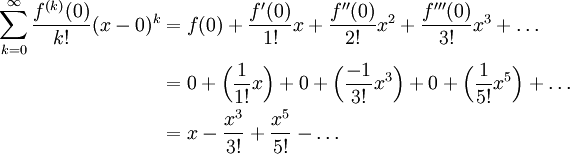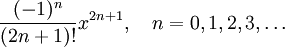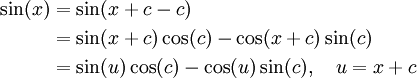# Maclaurin Expansion of sin(x)

Previous: Maclaurin Expansion of ex

Next: The Maclaurin Expansion of cos(x)

## Example

Find the Taylor series expansion for sin(x) at x = 0, and determine its radius of convergence.

## Complete Solution

Again, before starting this problem, we note that the Taylor series expansion at x = 0 is equal to the Maclaurin series expansion.

### Step 1: Find Coefficients

Let f(x) = sin(x). To find the Maclaurin series coefficients, we must evaluatefor k = 0, 1, 2, 3, 4, ...

Calculating the first few coefficients, a pattern emerges:The coefficients alternate between 0, 1, and -1. You should be able to, for the nth derivative, determine whether the nth coefficient is 0, 1, or -1.

### Step 2: Substitute Coefficients into Expansion

Thus, the Maclaurin series for sin(x) is### Step 3: Write the Expansion in Sigma Notation

From the first few terms that we have calculated, we can see a pattern that allows us to derive an expansion for the nth term in the series, which isSubstituting this into the formula for the Taylor series expansion, we obtainThe ratio test gives us:Because this limit is zero for all real values of x, the radius of convergence of the expansion is the set of all real numbers.

## Explanation of Each Step

### Step 1

Maclaurin series coefficients, ak can be calculated using the formula (that comes from the definition of a Taylor series)where f is the given function, and in this case is sin(x). In step 1, we are only using this formula to calculate the first few coefficients. We can calculate as many as we need, and in this case were able to stop calculating coefficients when we found a pattern to write a general formula for the expansion.

### Step 2

Step 2 was a simple substitution of our coefficients into the expression of the Taylor series.

### Step 3

A helpful step to find a compact expression for the nth term in the series, is to write out more explicitly the terms in the series that we have found:We have discovered the sequence 1, 3, 5, ... in the exponents and in the denominator of each term. We may then find a way to convert this sequence that we have discovered, into the sequence k=0, 1, 2, ... that appears in the final summation. The simple transform 2k+1 performs this transformation for us.

### Step 4

This step was nothing more than substitution of our formula into the formula for the ratio test. Because we found that the series converges for all x, we did not need to test the endpoints of our interval. If however we did find that the series only converged on an interval with a finite width, then we may need to take extra steps to determine the convergence at the boundary points of the interval.

### An Alternate Explanation

The following Khan Acadmey video provides a similar derivation of the Maclaurin expansion for sin(x) that you may find helpful.

Sine Taylor Series at 0
Derivation of the Maclaurin series expansion for sin(x).
This video can be found on the Kahn Academy website, and carries a Creative Commons copyright (CC BY-NC-SA 3.0).

## Possible Challenges

### What if we Need the Taylor Series of sin(x) at Some Other Point?

The Maclaurin series of sin(x) is only the Taylor series of sin(x) at x = 0. If we wish to calculate the Taylor series at any other value of x, we can consider a variety of approaches.

Suppose we wish to find the Taylor series of sin(x) at x = c, where c is any real number that is not zero. We could find the associated Taylor series by applying the same steps we took here to find the Macluarin series. That is, calculate the series coefficients, substitute the coefficients into the formula for a Taylor series, and if needed, derive a general representation for the infinite sum.

Another approach could be to use a trigonometric identity. Consider this approachThe functions cos(u) and sin(u) can be expanded in with a Maclaurin series, and cos(c) and sin(c) are constants. We will see the Maclaurin expansion for cosine on the next page.

### How Many Terms do I Need to Calculate?

It can be difficult to find an expression for the nth term in the series that allows us to write out a compact expression for an infinite sum. In our example here, we only calculated three terms. It may be helpful in other problems to write out a few more terms to find a useful pattern.

## Summary

To summarize, we found the Macluarin expansion of the sine function.

The Maclaurin Expansion of sin(x)
The Maclaurin series expansion for sin(x) is given byThis formula is valid for all real values of x.

Previous: Maclaurin Expansion of ex

Next: The Maclaurin Expansion of cos(x)

### 1 Response to Maclaurin Expansion of sin(x)

1.David Lowry-Duda says:

I think this is a nice and clear post. My students have trouble with Taylor series each time I teach it, and there is something about sine that makes it the appropriate jumping point.

As a sort of play or alternate viewing, I wrote up with a different derivation (sort of a heuristical derivation) of the Taylor series for sine. I write it here only because I think it plays against this exposition nicely.